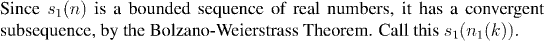Analysis WebNotes
arrow_back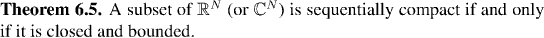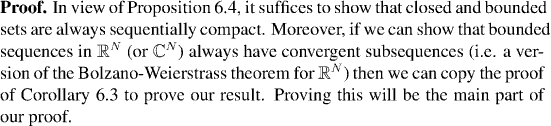Although the idea of the proof is pretty simple, it involves some rather fierce notation. Click here for the proof in R2, which involves the key ideas, but without the need for complicated notation.

Some Notation: In order to make the proof of the theorem go a little more smoothly we need some notation.

If xn is a sequence of points in the set X, let's consider the question: what sort of thing is (xn)?

The sequence provides a correspondence between the set of natural numbers and elements of X, so the answer is that really the sequence is no more or less than a function from the natural numbers to X. In fact, in general, that's a any sequence is; a function from the natural numbers into some set. From this point of view it makes sense to write x(n) instead of xn, or even to write;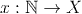So why do we generally use a different notation for sequences than for all other kinds of functions? Just the force of tradition, together with the fact that the notation does work well for most uses of sequences. In the present situation it makes more sense to write our sequences as functions x(n).

What is a subsequence going to be in this new notation? A subsequence is just a change of variable, or composition of functions, to get x(n(k)), where n(k) is a function of the natural numbers into themselves which must be strictly increasing.

Now we'll use this notation to continue the proof of Theorem 6.5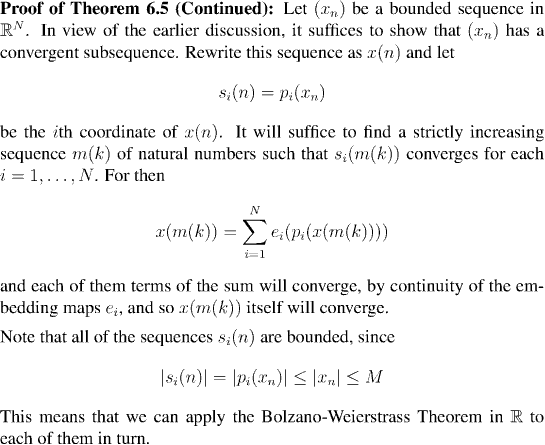We construct the appropriate subsequence in a series of steps. Click on each step in oder to proceed to the next: